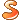# 强化学习系列之二:模型相关的强化学习

上一章我们介绍了强化学习的基本假设——马尔科夫决策过程 (Markov Decision Process)。本文将介绍模型相关的强化学习算法。

有的时候，我们完全知道问题背后的马尔科夫决策过程；有的时候，我们不知道问题背后的马尔科夫决策过程 (主要指我们不知奖励函数 (R_{s,a}) 和转移概率 (P_{s,a}^{s’}) 的全貌)。根据马尔科夫决策过程是否可知，强化学习可以分为两类: 模型相关 (Model-based) 和模型无关 (Model-free)。模型相关是我们知道整个马尔科夫决策过程。模型无关则是我们不知道马尔科夫决策过程，需要系统进行探索。今天我们先介绍比较简单的模型相关强化学习。

本文还是以机器人寻找金币为例子。如下图所示，一个机器人出发寻找金币。找到金币则获得奖励 1，碰到海盗则损失 1。找到金币或者碰到海盗则机器人停止。图中不同位置为状态，因此状态集合 S = {1,…,5}。机器人采取的动作是向东南西北方向走，因此A={‘n’,’e’,’s’,’w’}。转移概率方面，当机器人碰到墙壁，则会停在原来的位置；当机器人找到金币时获得奖励 1，当碰到海盗则损失 1, 其他情况不奖励也不惩罚。因此除了 $$R_{1,s} = -1$$, $$R_{3,s} = 1$$，$$R_{5,s} = -1$$ 之外，其他情况$$R_{,}=0$$。$$\gamma$$衰减因子等于 0.8。

class Policy_Value:
def __init__(self, grid_mdp):
//self.v[state] 等于状态 state 的价值。
self.v  = [ 0.0 for i in xrange(len(grid_mdp.states) + 1)]

//self.pi[state] 等于策略在状态 state 下采取的动作
self.pi = dict()
for state in grid_mdp.states:
if state in grid_mdp.terminal_states: continue
self.pi[state] = grid_mdp.actions[ 0 ]


### 1. 策略迭代

模型相关的强化学习算法主要有：策略迭代 (Policy Iteration) 和价值迭代 (Value Iteration)。策略迭代的主要思想是这样的。先随机初始化一个策略 (\pi_0)，计算这个策略下每个状态的价值 (\pmb{v}_0)；根据这些状态价值得到新策略 (\pi_1)；计算新策略下每个状态的价值 (\pmb{v}_1) … 直到收敛。计算一个策略下每个状态的价值，被称为策略评估 (Policy Evaluation)；根据状态价值得到新策略，被称为策略改进 (Policy Improvement)。

#### 1.1 策略评估

策略评估利用了贝尔曼等式。根据贝尔曼等式，一个状态的价值和它后续状态的价值有关。因此我们用后续状态价值 (v(s’)) 去更新当前状态的价值 (v(s))。策略评估遍历所有状态，按照下面公式更新其价值。

\begin{eqnarray}
v(s) = \sum_{a \in A}\pi(s,a)(R_{s,a}+\gamma \sum_{s’ \in S}T_{s,a}^{s’}v_{t}(s’)) \nonumber
\end{eqnarray}

由于状态之间是可以相互转移的，比如 s’ 可以是 s 的后续状态，但反过来 s 也可以是 s’ 后续状态。如果只遍历并更新一遍状态价值，状态价值并不是当前策略的价值。为此我们多次遍历并更新状态价值。策略评估的代码 (代码在这个链接下的 policy_iteration.py 中)。

// grid_mdp 是机器人找金币问题的马尔科夫决策过程
def policy_evaluate(self, grid_mdp):
for i in xrange(1000):

delta = 0.0
for state in grid_mdp.states:
if state in grid_mdp.terminal_states: continue
// 当前策略输出动作
action        = self.pi[state]
// t 表示是否到了终止状态
// s 表示状态 state 下执行动作 action 后的状态
// r 表示奖励
t, s, r       = grid_mdp.transform(state, action)
//更新状态价值
new_v         = r + grid_mdp.gamma * self.v[s]
delta        += abs(self.v[state] - new_v)
self.v[state] = new_v

if delta < 1e-6:
break;


#### 1.2 策略改进

根据状态价值得到新策略，被称为策略改进。对于一个状态s，让策略选择一个动作a，使得当前状态的价值 (R_{s,a}+\gamma \sum_{s’ \in S}T_{s,a}^{s’}v(s’) ) 最大，即
\begin{eqnarray}
\pi_{i+1}(s,a) =
\left{\begin{matrix}
1 & a = argmax_{a}R_{s,a}+\gamma \sum_{s’ \in S}T_{s,a}^{s’}v(s’) \
0 & a \neq argmax_{a}R_{s,a}+\gamma \sum_{s’ \in S}T_{s,a}^{s’}v(s’)
\end{matrix}\right. \nonumber
\end{eqnarray}

可以向你保证，这样得到的新策略一定是优于旧策略的，即(v_{\pi_{i+1}}(s) \geq v_{\pi_{i}}(s), \forall s) (上一篇文章最后一节介绍的策略偏序关系)。具体证明就不详细列出了，说下大致思路：我们用策略评估计算新策略的状态价值；第一个要更新的状态是 s1，因为新策略选择价值最大的动作，因此新策略使得 v(s1) 上升；如果之前的更新都是使得价值上升，那么更新当前状态 sk 时，新策略还是使得价值上升；即使一个状态被多次更新，价值都是上升的。这样新策略优于旧策略。策略改进的代码如下 (代码在这个链接下的 policy_iteration.py 中)

def policy_improve(self, grid_mdp):

for state in grid_mdp.states:
if state in grid_mdp.terminal_states: continue

a1      = grid_mdp.actions
t, s, r = grid_mdp.transform( state, a1 )
v1      = r + grid_mdp.gamma * self.v[s]

//找到当前状态的价值最大的动作 a1
for action in grid_mdp.actions:
t, s, r = grid_mdp.transform( state, action )
if v1 < r + grid_mdp.gamma * self.v[s]:
a1 = action
v1 = r + grid_mdp.gamma * self.v[s]
//更新策略
self.pi[state] = a1


策略迭代交替执行策略评估和策略改进直到收敛，从而得到最优策略了。下图是策略迭代在机器人找金币问题中找到的最优解。

### 2. 价值迭代

看完策略迭代，我们发现策略迭代计算起来挺麻烦的，代码实现起来也麻烦。我们能不能把策略评估和策略改进结合得更紧密一些。一个状态下选择动作一旦确定，立刻按照这个动作计算当前状态的价值。这个做法其实就是价值迭代。价值迭代的代码如下所示 (代码在这个链接下的 value_iteration.py 中)。

def value_iteration(self, grid_mdp):
for i in xrange(1000):

delta = 0.0;
for state in grid_mdp.states:

if state in grid_mdp.terminal_states: continue

a1      = grid_mdp.actions
t, s, r = grid_mdp.transform( state, a1 )
v1      = r + grid_mdp.gamma * self.v[s]

for action in grid_mdp.actions:
t, s, r = grid_mdp.transform( state, action )
if v1 < r + grid_mdp.gamma * self.v[s]:
a1 = action
v1 = r + grid_mdp.gamma * self.v[s]

delta         += abs(v1 - self.v[state])
self.pi[state] = a1
self.v[state]  = v1;

if delta <  1e-6:
break;



价值迭代一直执行直到收敛，从而获得最优策略。下图是价值迭代在机器人找金币问题中找到的最优解，和策略迭代找到的是一样的。

### 3. 总结性结尾（好魔性的标题）

本文介绍了模型相关的强化学习。模型相关的强化学习是指马尔科夫决策过程可知情况下的强化学习，其中策略迭代和价值迭代是主要的两种算法。本文代码可以在 Github 上找到，欢迎有兴趣的同学帮我挑挑毛病。强化学习系列的下一篇文章将介绍模型无关的强化学习，将涉及到 TD 、SARSA 和 Q-Learning 等著名算法。

文章结尾欢迎关注我的公众号 AlgorithmDog，每周日的更新就会有提醒哦~

### 《强化学习系列之二:模型相关的强化学习》有 17 条评论

1.杨宇轩说：

期待下一篇，有示例和实际代码更容易理解了。谢谢博主分享

2.jeff说：

楼主，你好，我想问一下你示例代码是自己写的吗？还是说有相关的资料。想知道你学习RL的资料~[挤眼]求推荐~

•上微博的猫V说：

代码是自己写的哈。学习资料是 David Silver 的课程，地址http://www0.cs.ucl.ac.uk/staff/d.silver/web/Teaching.html

•jeff说：

谢谢啦~

3.学名马铃薯说：

我看价值迭代处的循环相反的传值需要再次计算，可不可以用马尔可夫随机场去处理迭代

•上微博的猫V说：

我不是很了解马尔可夫随机场哈。模型相关的强化学习用于讲解概念，实际用处倒是不大，所以也没有必要优化太多~

4.说：

似乎没有体现转移概率？

•上微博的猫V说：

找金币的例子中，转移概率确实不突出。你可以理解为T(1,’s’,6) = 1, T(1,’s’,除了6状态) = 0。

5.闻风丧当说：

博主你好，感谢你的教程！在你的例子中，关于转移概率可以这样理解么？就是一旦动作决定了，转移函数的结果就是一个确定值，而不是一个分布。

•上微博的猫V说：

恩恩，是的。你可以理解为p(1,’e’,2) = 1,p(1,’e’,3) = 0等等。

•庞小文说：

博主你好呀，所以我还有个问题，就是既然转移函数是确定的，那policy_evaluate这个函数里迭代1000次在这个程序里不是必须的？谢谢博主～～

•Wolfgang说：

这里是必须的吧，因为计算Ut要达到收敛才行。

6.Wolfgang说：

博主你好，为什么improve function 里的 a1 = grid_mdp.actions 每次都要是actions[0】呢，而不是当前pi下在该状态的action，也就是 self.pi[state] .

7.韦峰说：

a1 = grid_mdp.actions t, s, r = grid_mdp.transform( state, a1 ) v1 = r + grid_mdp.gamma * self.v这个是不是和他下面的for action in grid_mdp.actions: t, s, r = grid_mdp.transform( state, action ) if v1 < r + grid_mdp.gamma * self.v: a1 = action v1 = r + grid_mdp.gamma * self.v重复了？

8.匿名说：

代码失效了，求博主代码

•匿名说：

不是有 github 链接嘛？

9.ArtsCrafts说：

你好 请问策略评估公式中的T_{s,a}^{s’} 表示的是是从 状态 s 转移到 s’ 的概率嘛？